# Power of a number Using Recursion in C

## Power of a given number Using Recursion in C – Exponent (mn):

In this article, I am going to discuss the Power of a given number using Recursion in C Language with Examples. Please read our previous article where we discussed the Factorial of a given number using Recursion in C Language with Examples.

##### Understanding Exponent Function

Here, first, we will talk about exponent function i.e. mn that is raising m for n times that is multiplying m for n number of times. Below is the mathematical expression of power: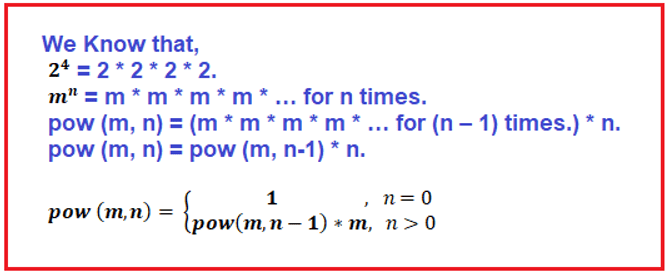We have two ways to define exponent recursive function are as follows:

1. The first method requires more numbers of multiplication.
2. The second method requires less number of multiplication.
##### Method 1:

In the following example, we created a function pow which takes two parameters v (the number) and e (the exponent). In the next step, we define our base condition (where the function terminates or ends) which is if e == 0. If it satisfies then return 1 otherwise return pow(v, e – 1) * v.

In this pow function, the value will be returned at returning time from the recursive calls. This function will terminate at e == 0. And when it terminates it returns 1, from there it starts multiplying this 1 with function parameter v, i.e., 2 * 2 * 2 * 2. And in this manner, our recursive function pow will calculate the exponent of the given value. If this is not clear at the moment don’t worry once we discuss the tracing tree of this example, then definitely you understand this recursive function.##### Tracing Tree of the above Program:

Now, Let’s jump on the Tracing Tree where we will understand each and every step.

##### Step1: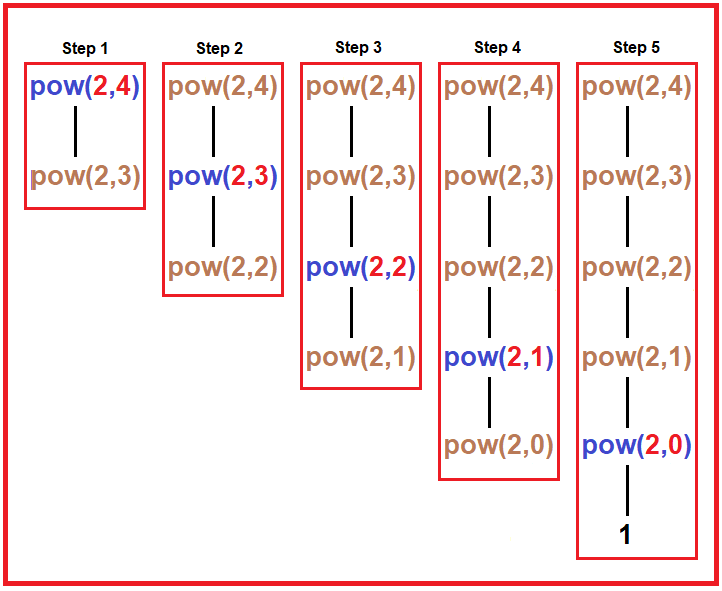Tree – In the above diagram, there are 5 steps. In every step, we have shown every recursive call of the pow function. As shown in the diagram, we passed 2 and 4 as a parameter.

1. In 1st step, it checks if 4 == 0? No, then it goes to the else part which returns pow (2, 4 – 1) * 2. Here we can’t perform multiplication, first, it has to finish pow (2, 4 – 1) call then only multiplication will perform.
2. In 2nd step, it checks if 3 == 0? No, then it goes to the else part which returns pow (2, 3 – 1) * 2. Here also we will have to first calculate the result of pow (2, 3 – 1) call then only multiplication will perform.
3. In 3rd step, it checks if 2 == 0? No, then it goes to the else part which returns pow (2, 2 – 1) * 2. we will have to first calculate the result of pow (2, 2 – 1) call then only multiplication will perform.
4. In 4th step, it checks if 1 == 0? No, then it goes to the else part which returns pow (2, 1 – 1) * 2. we will have to first calculate the result of pow (2, 1 – 1) call then only multiplication will perform.
5. In 5th step, it checks if 0 == 0? Yes, then it returns 1. And from here multiplication starts, returning time from recursive calls. Now it will multiply the result of each recursive call with the 2 and at the final call, it will finally return the calculated value of pow of the given value.
##### Step2: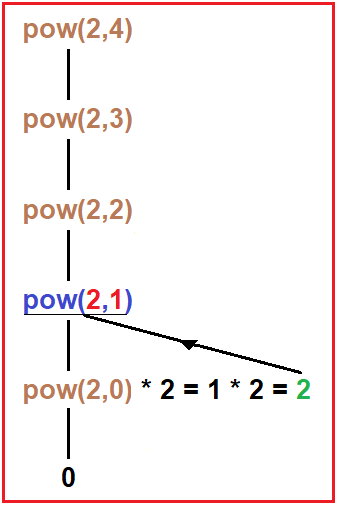Tree – Now as we discussed in the previous step when the base condition (e == 0) true then it will return 1. And this 1 will return to the previous call and be multiplied by the given value of v (2) as shown in the diagram, here the result of the multiplication is 1 which further returns to the previous recursive call. Here multiplied value is 2 which return to pow (2, 1) call.

##### Step3: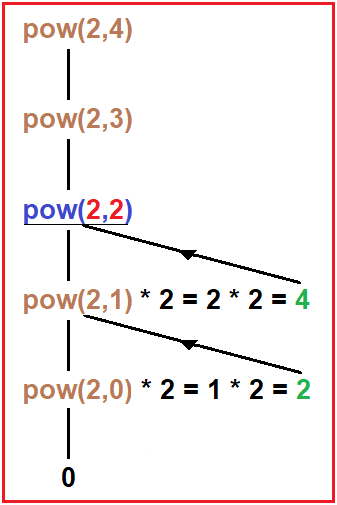Tree – Now the multiplied value is 4 which returns to pow (2, 2) call and further multiplied by the value of v i.e., 2.

##### Step4: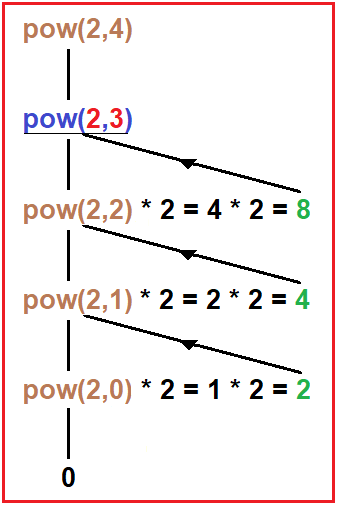Tree – Now here the multiplication takes place between 4 and 2 and the calculated value is 8 which returns to the pow (2, 3) call.

##### Step5: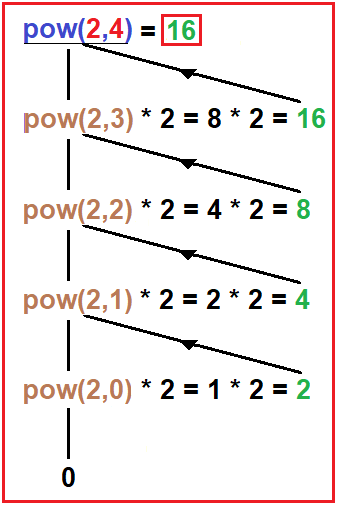Tree – Now the final calculated value Is 16 which pow (2, 4) returns to that place where we have called this inside the main function. In our current example, we call this function in the print statement. Now, as the function return the final value it will print on the output screen.

##### Complete Code:
```#include <stdio.h>
int power(int v, int e) {
if (e == 0)
return 1;
return power(v, e - 1) * v;
}

int main() {
int v = 2, e = 4;
printf("%d", power(v, e));
}
```

Output: 16

Time Complexity: O(e)

Note: Total number of multiplications performed: e times

##### Method 2:

We will modify the previous recursive function so that it computes with less number of multiplications. Then the function takes less time and less memory in the stack. We can write 24 as 24 = (22)2. The above statement state if we perform one multiplication on the same value the power will reduce by half. And suppose we have 29 then we can write it as 29 = 2 * (22)4

In the above expression, the exponent of 2 is odd which is 9. So, in this situation, we separate one 2 and then half the power. So, there can be two conditions:

1. If power is even – then directly half the power.
2. If power is odd – then first separate one value from power and add on multiplication with that number, then half the power.

The following piece of code does the above two things.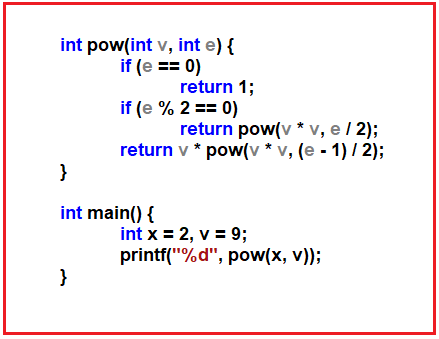##### Tracing Tree of the above Recursion Program:

Now, Let’s jump on the Tracing Tree where we will understand each and every step.

##### Step1: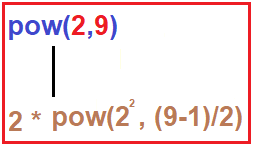Tree: We pass 2 as a number and 9 as the exponent in the pow function. Then it checks its base condition which is if 9 == 0? No, moving to next if condition which checks for if e is even? No, then it goes to the else part which returns 2 * pow (2 * 2, (9 – 1)/2) which equals 2 * pow (22, 4).

##### Step2: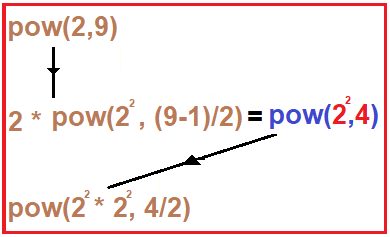Tree: Here it checks its base condition which is if 4 == 0? No, moving to next if condition which checks for if e is even? Yes, which return pow (22 * 22, 4/2) which equals pow (24, 2).

##### Step3: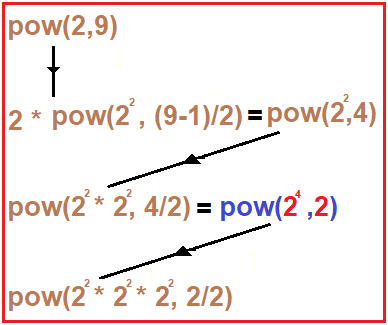Tree: Here it checks its base condition which is if 2 == 0? No, moving to next if condition which checks for if e is even? Yes, which return pow (22 * 22 * 22, 2/2) which equals pow (28, 1).

##### Step4: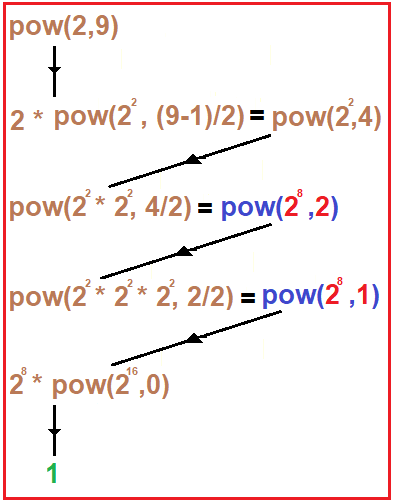Tree: It checks its base condition if 1 == 0? No, moving to next if condition which checks for if e is even? No, then it goes to else part which return 28 * pow (28 * 28, (1 – 1)/2) which equals to 28 * pow (216, 0), In pow (216, 0) it will check for if 0 == 0? Yes, then from here it will return 1.

##### Step5: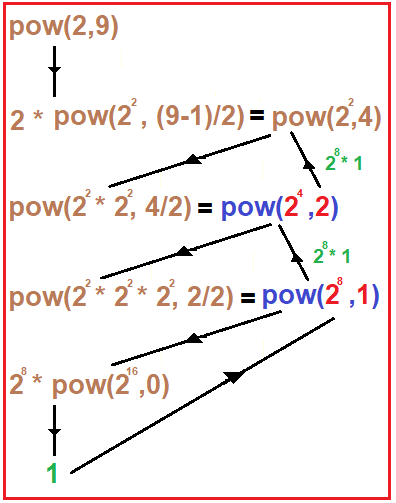Tree: In our previous step, 1 is returned to pow (28, 1). From this call 28 * 1 will return to pow (24, 2). As there is no pending multiplication then this also returns 28 * 1 to pow (22, 4).

##### Step6: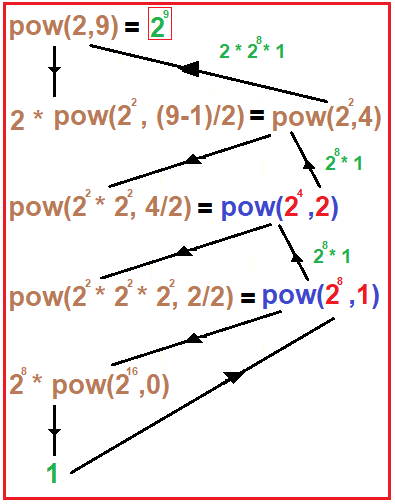Tree: In our previous step, 28 * 1 to pow (22, 4). Now, here is pending multiplication which is 2 * to pow (22, 4). And this will return 2 * 28 * 1 to pow (2, 9). The final calculated value is 29 which is 512.

The above method requires less number of multiplication in comparison with the previous recursive method.

##### Complete Code:
```#include <stdio.h>
int power(int v, int e) {
if (e == 0)
return 1;
if (e % 2 == 0)
return power(v * v, e / 2);
return v * power(v * v, (e - 1) / 2);
}

int main() {
int x = 2, v = 9;
printf("%d", power(x, v));
}
```

Output: 512

In the next article, I am going to discuss Taylor Series using Recursion in C Language with Example. Here, in this article, I try to explain the Power of a given number in C Language using Recursion with Example and I hope you enjoy this Power of a given number using Recursion in C Language with Example article.